# sparse inverse covariance (PDF)

### File information

This PDF 1.5 document has been generated by TeX / pdfTeX-1.40.17, and has been sent on pdf-archive.com on 19/01/2017 at 11:06, from IP address 185.127.x.x. The current document download page has been viewed 721 times.
File size: 651 KB (7 pages).
Privacy: public file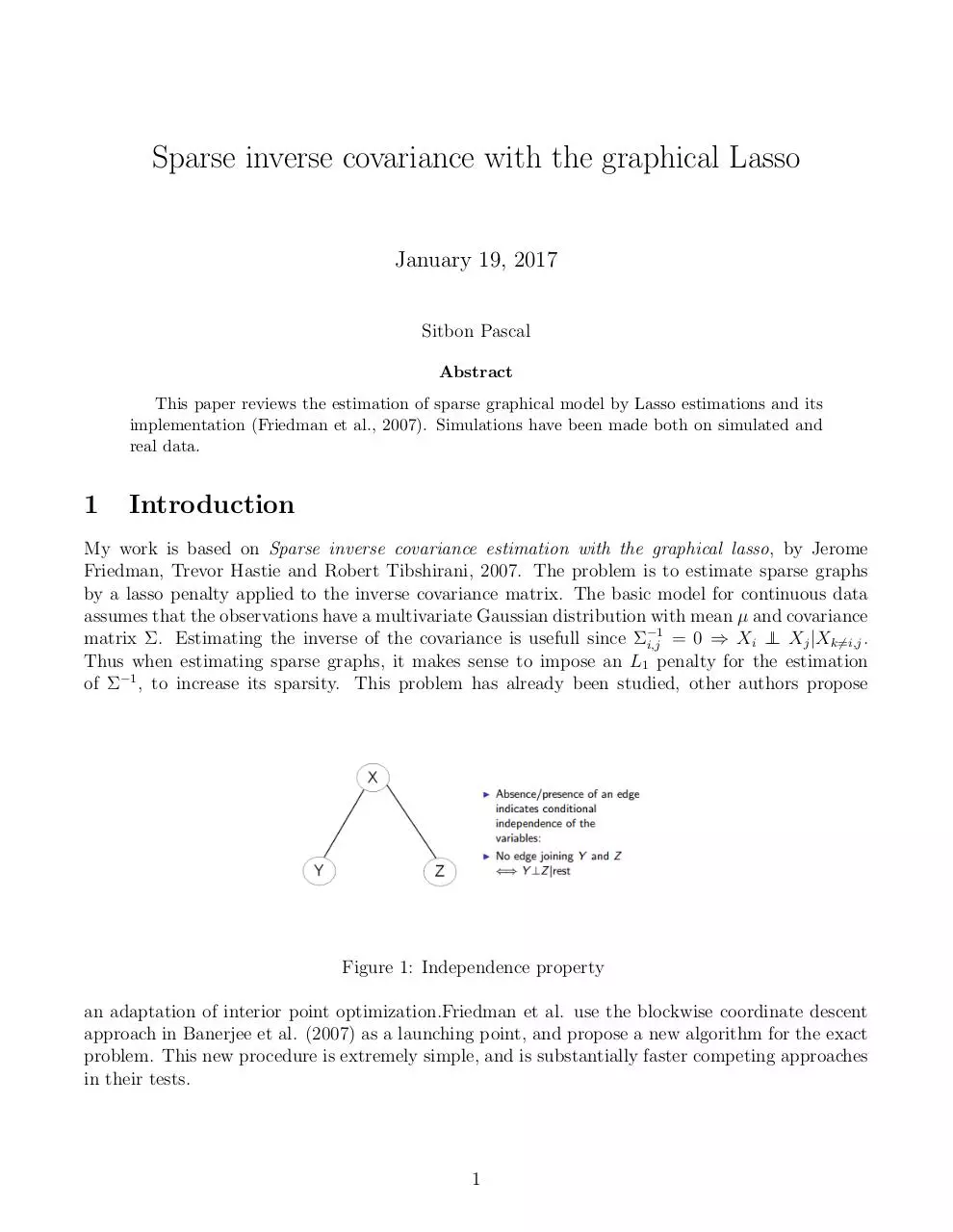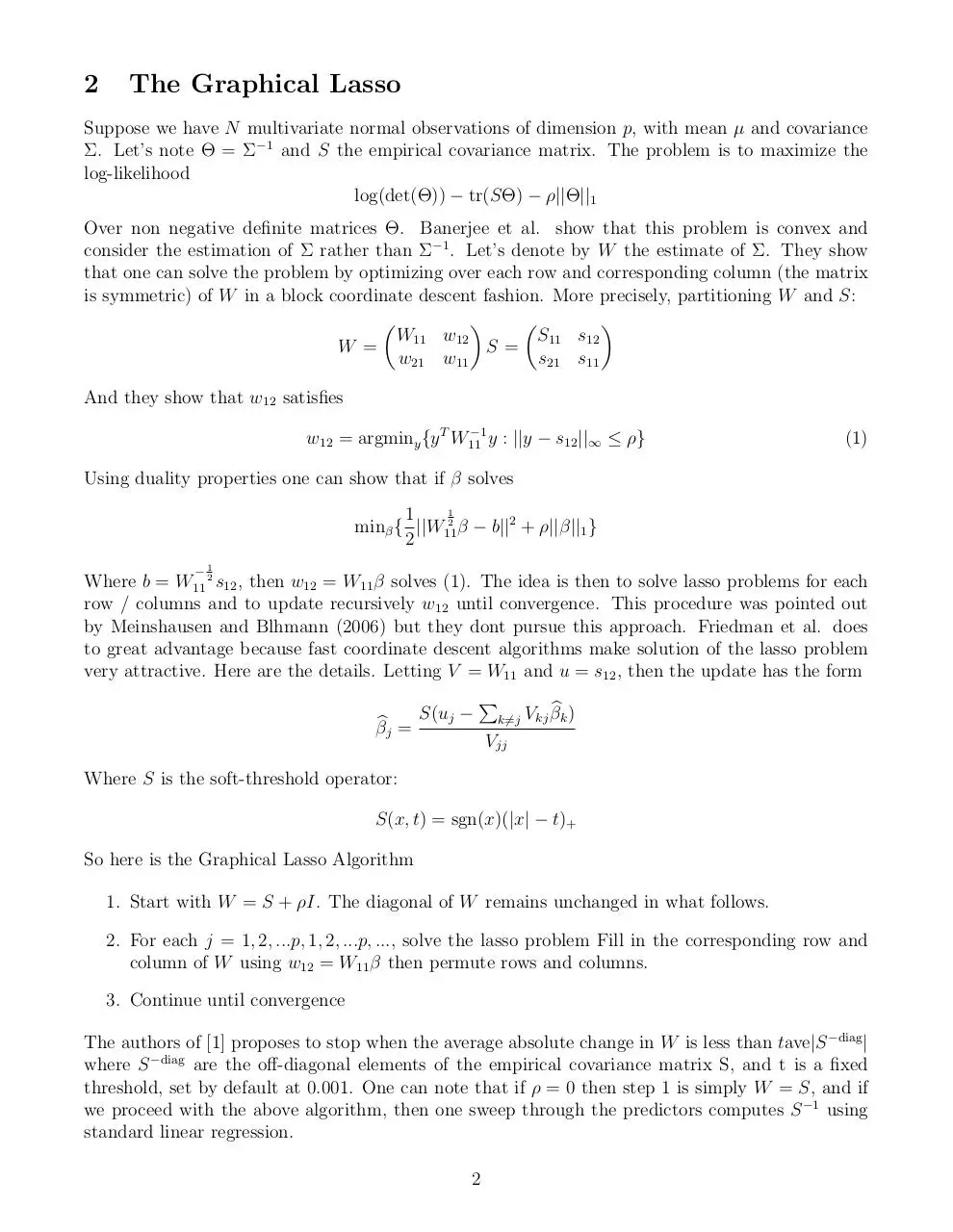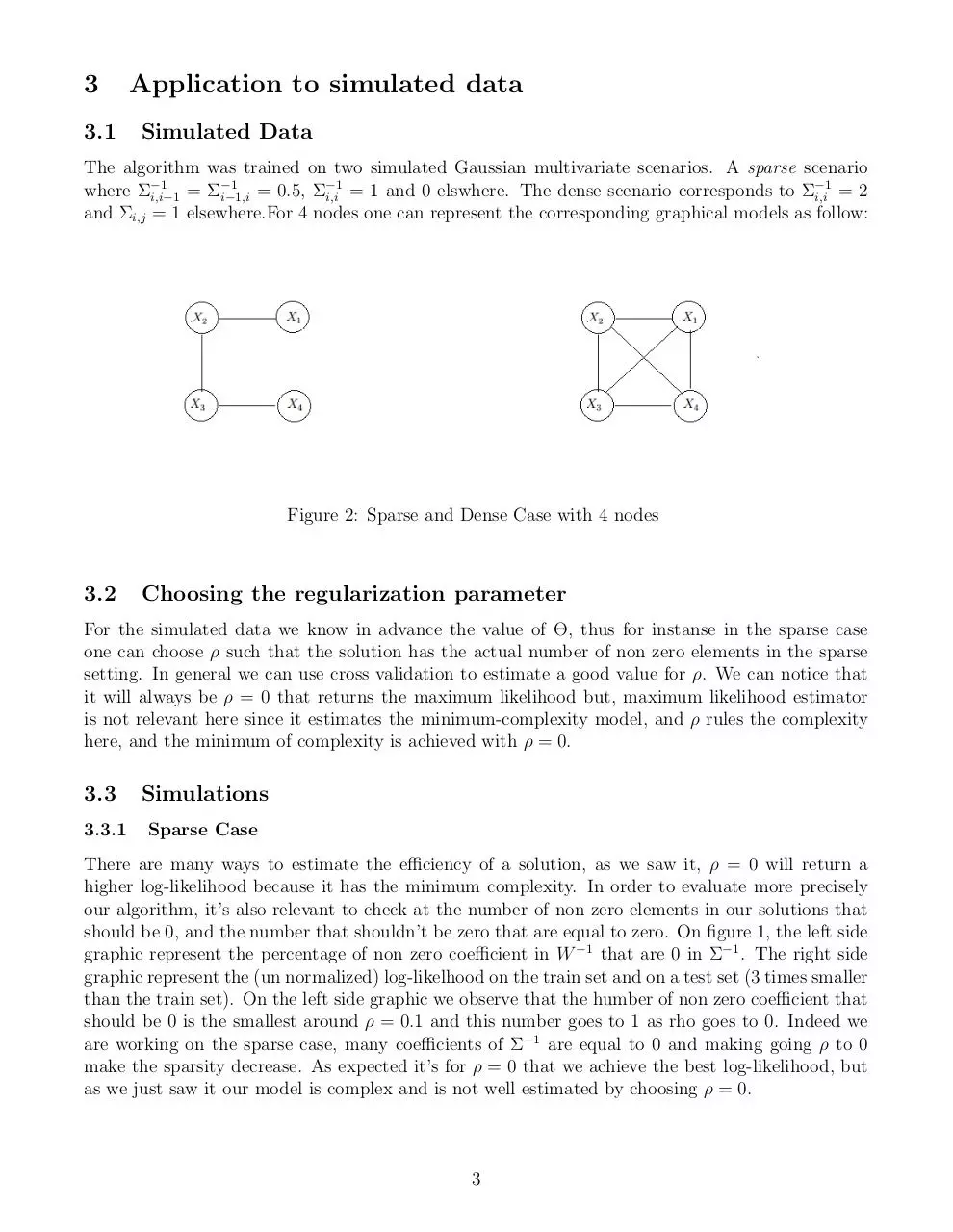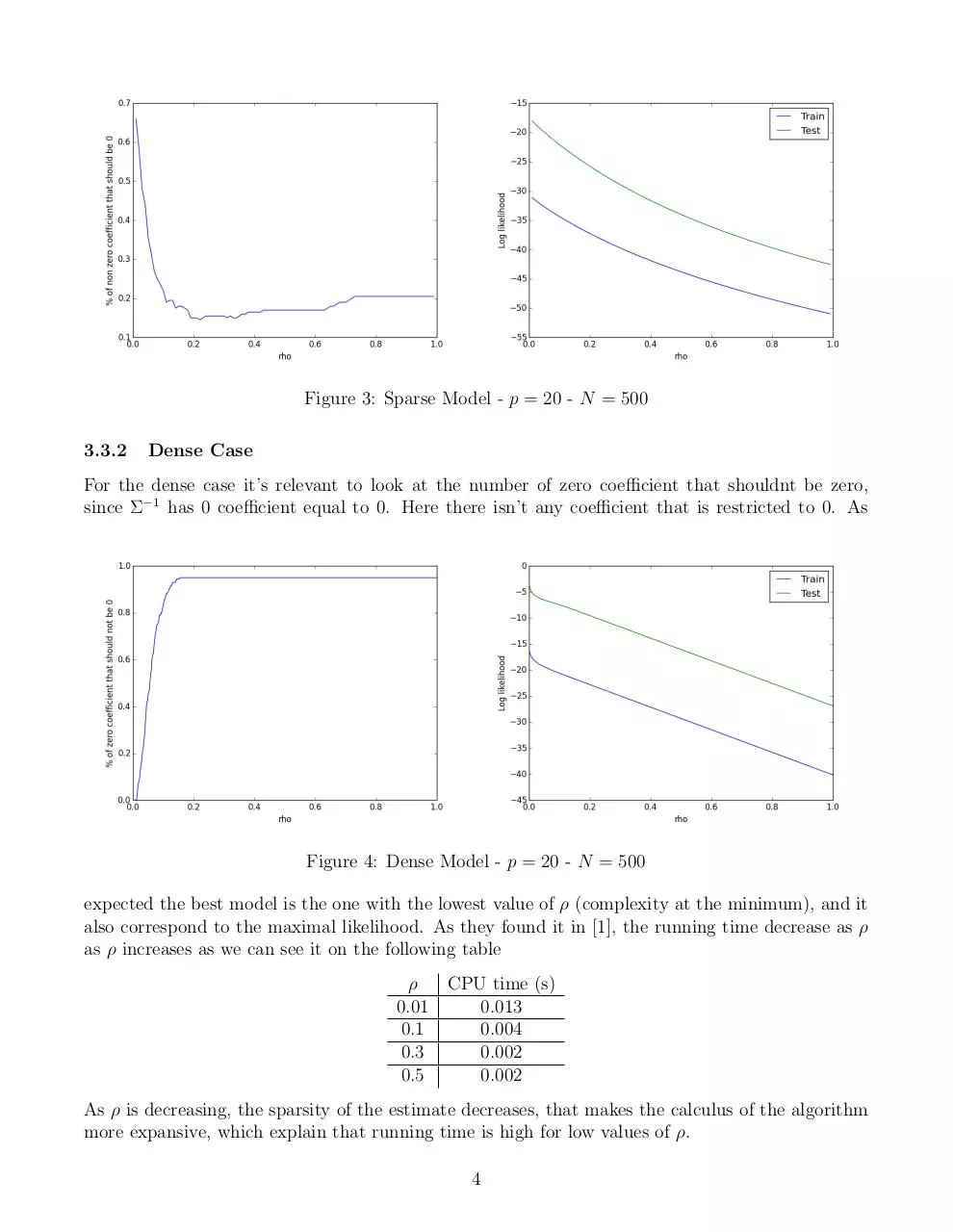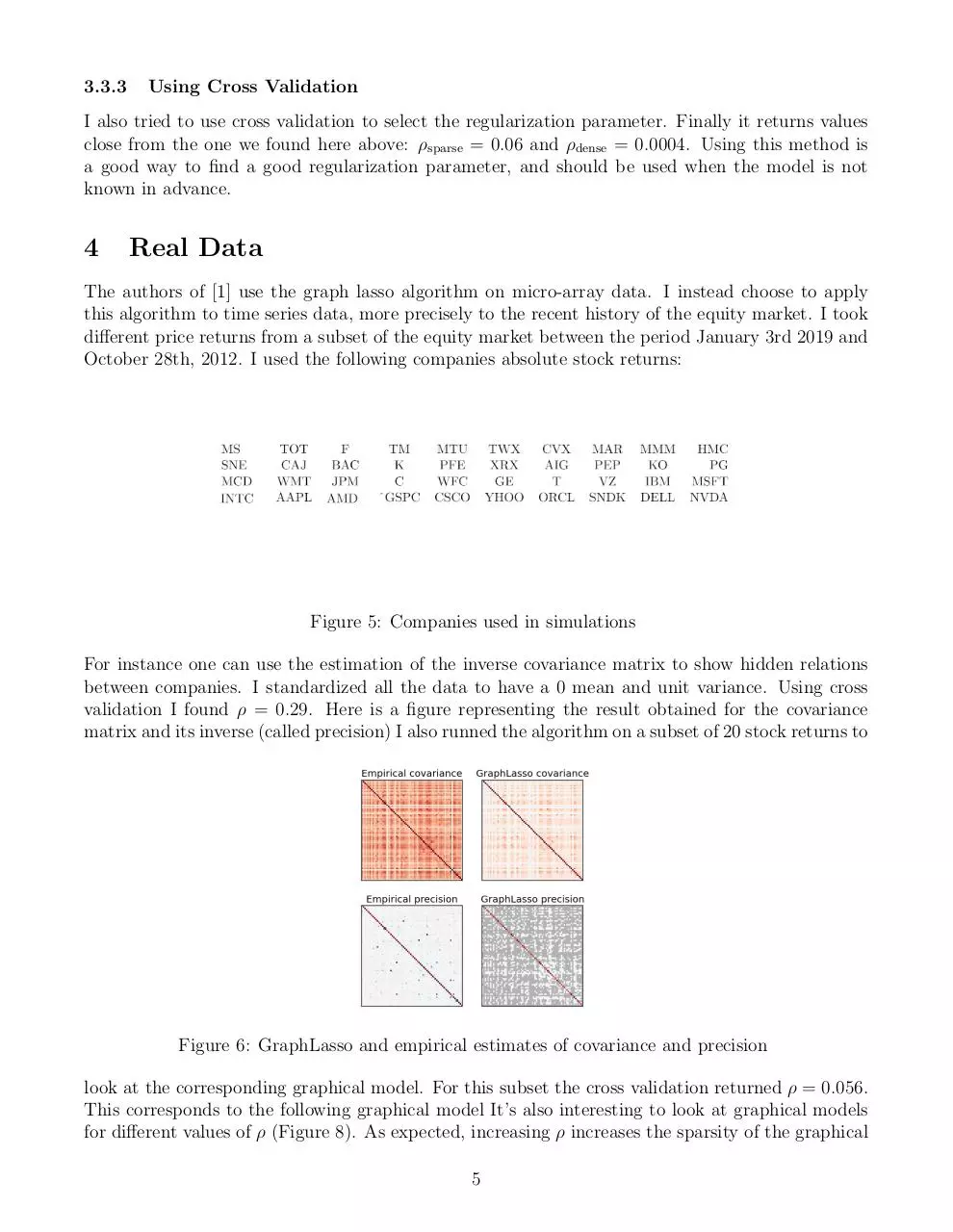### File preview

Sparse inverse covariance with the graphical Lasso
January 19, 2017
Sitbon Pascal
Abstract
This paper reviews the estimation of sparse graphical model by Lasso estimations and its
implementation (Friedman et al., 2007). Simulations have been made both on simulated and
real data.

1

Introduction

My work is based on Sparse inverse covariance estimation with the graphical lasso, by Jerome
Friedman, Trevor Hastie and Robert Tibshirani, 2007. The problem is to estimate sparse graphs
by a lasso penalty applied to the inverse covariance matrix. The basic model for continuous data
assumes that the observations have a multivariate Gaussian distribution with mean µ and covariance
matrix Σ. Estimating the inverse of the covariance is usefull since Σ−1
⊥ Xj |Xk6=i,j .
i,j = 0 ⇒ Xi ⊥
Thus when estimating sparse graphs, it makes sense to impose an L1 penalty for the estimation
of Σ−1 , to increase its sparsity. This problem has already been studied, other authors propose

Figure 1: Independence property
an adaptation of interior point optimization.Friedman et al. use the blockwise coordinate descent
approach in Banerjee et al. (2007) as a launching point, and propose a new algorithm for the exact
problem. This new procedure is extremely simple, and is substantially faster competing approaches
in their tests.

1

2

The Graphical Lasso

Suppose we have N multivariate normal observations of dimension p, with mean µ and covariance
Σ. Let’s note Θ = Σ−1 and S the empirical covariance matrix. The problem is to maximize the
log-likelihood
log(det(Θ)) − tr(SΘ) − ρ||Θ||1
Over non negative definite matrices Θ. Banerjee et al. show that this problem is convex and
consider the estimation of Σ rather than Σ−1 . Let’s denote by W the estimate of Σ. They show
that one can solve the problem by optimizing over each row and corresponding column (the matrix
is symmetric) of W in a block coordinate descent fashion. More precisely, partitioning W and S:




W11 w12
S11 s12
W =
S=
w21 w11
s21 s11
And they show that w12 satisfies
−1
w12 = argminy {y T W11
y : ||y − s12 ||∞ ≤ ρ}

(1)

Using duality properties one can show that if β solves
1
1
minβ { ||W112 β − b||2 + ρ||β||1 }
2

−1

Where b = W11 2 s12 , then w12 = W11 β solves (1). The idea is then to solve lasso problems for each
row / columns and to update recursively w12 until convergence. This procedure was pointed out
by Meinshausen and Blhmann (2006) but they dont pursue this approach. Friedman et al. does
to great advantage because fast coordinate descent algorithms make solution of the lasso problem
very attractive. Here are the details. Letting V = W11 and u = s12 , then the update has the form
P
S(uj − k6=j Vkj βbk )
βbj =
Vjj
Where S is the soft-threshold operator:
S(x, t) = sgn(x)(|x| − t)+
So here is the Graphical Lasso Algorithm
1. Start with W = S + ρI. The diagonal of W remains unchanged in what follows.
2. For each j = 1, 2, ...p, 1, 2, ...p, ..., solve the lasso problem Fill in the corresponding row and
column of W using w12 = W11 β then permute rows and columns.
3. Continue until convergence
The authors of  proposes to stop when the average absolute change in W is less than tave|S −diag |
where S −diag are the off-diagonal elements of the empirical covariance matrix S, and t is a fixed
threshold, set by default at 0.001. One can note that if ρ = 0 then step 1 is simply W = S, and if
we proceed with the above algorithm, then one sweep through the predictors computes S −1 using
standard linear regression.
2

3
3.1

Application to simulated data
Simulated Data

The algorithm was trained on two simulated Gaussian multivariate scenarios. A sparse scenario
−1
−1
−1
where Σ−1
i,i−1 = Σi−1,i = 0.5, Σi,i = 1 and 0 elswhere. The dense scenario corresponds to Σi,i = 2
and Σi,j = 1 elsewhere.For 4 nodes one can represent the corresponding graphical models as follow:

Figure 2: Sparse and Dense Case with 4 nodes

3.2

Choosing the regularization parameter

For the simulated data we know in advance the value of Θ, thus for instanse in the sparse case
one can choose ρ such that the solution has the actual number of non zero elements in the sparse
setting. In general we can use cross validation to estimate a good value for ρ. We can notice that
it will always be ρ = 0 that returns the maximum likelihood but, maximum likelihood estimator
is not relevant here since it estimates the minimum-complexity model, and ρ rules the complexity
here, and the minimum of complexity is achieved with ρ = 0.

3.3

Simulations

3.3.1

Sparse Case

There are many ways to estimate the efficiency of a solution, as we saw it, ρ = 0 will return a
higher log-likelihood because it has the minimum complexity. In order to evaluate more precisely
our algorithm, it’s also relevant to check at the number of non zero elements in our solutions that
should be 0, and the number that shouldn’t be zero that are equal to zero. On figure 1, the left side
graphic represent the percentage of non zero coefficient in W −1 that are 0 in Σ−1 . The right side
graphic represent the (un normalized) log-likelhood on the train set and on a test set (3 times smaller
than the train set). On the left side graphic we observe that the humber of non zero coefficient that
should be 0 is the smallest around ρ = 0.1 and this number goes to 1 as rho goes to 0. Indeed we
are working on the sparse case, many coefficients of Σ−1 are equal to 0 and making going ρ to 0
make the sparsity decrease. As expected it’s for ρ = 0 that we achieve the best log-likelihood, but
as we just saw it our model is complex and is not well estimated by choosing ρ = 0.

3

Figure 3: Sparse Model - p = 20 - N = 500
3.3.2

Dense Case

For the dense case it’s relevant to look at the number of zero coefficient that shouldnt be zero,
since Σ−1 has 0 coefficient equal to 0. Here there isn’t any coefficient that is restricted to 0. As

Figure 4: Dense Model - p = 20 - N = 500
expected the best model is the one with the lowest value of ρ (complexity at the minimum), and it
also correspond to the maximal likelihood. As they found it in , the running time decrease as ρ
as ρ increases as we can see it on the following table
ρ
CPU time (s)
0.01
0.013
0.1
0.004
0.3
0.002
0.5
0.002
As ρ is decreasing, the sparsity of the estimate decreases, that makes the calculus of the algorithm
more expansive, which explain that running time is high for low values of ρ.
4

3.3.3

Using Cross Validation

I also tried to use cross validation to select the regularization parameter. Finally it returns values
close from the one we found here above: ρsparse = 0.06 and ρdense = 0.0004. Using this method is
a good way to find a good regularization parameter, and should be used when the model is not

4

Real Data

The authors of  use the graph lasso algorithm on micro-array data. I instead choose to apply
this algorithm to time series data, more precisely to the recent history of the equity market. I took
different price returns from a subset of the equity market between the period January 3rd 2019 and
October 28th, 2012. I used the following companies absolute stock returns:

Figure 5: Companies used in simulations
For instance one can use the estimation of the inverse covariance matrix to show hidden relations
between companies. I standardized all the data to have a 0 mean and unit variance. Using cross
validation I found ρ = 0.29. Here is a figure representing the result obtained for the covariance
matrix and its inverse (called precision) I also runned the algorithm on a subset of 20 stock returns to

Figure 6: GraphLasso and empirical estimates of covariance and precision
look at the corresponding graphical model. For this subset the cross validation returned ρ = 0.056.
This corresponds to the following graphical model It’s also interesting to look at graphical models
for different values of ρ (Figure 8). As expected, increasing ρ increases the sparsity of the graphical
5

Figure 7: Graphical model ρ = 0.056
model. Looking at the edges that are the largest indicates hidden relation between variables. The
absence of edge between two companies means that they evolve independently knowing the evolution
of the other companies. An idea to explore would be to find out some ”networks” that evolve

Figure 8: Graphical model for ρ = 0.35
independently from the rest, and to find out hidden links between companies of these networks).
We can also

6

5

Conclusion

Estimating sparse graphical model is still studied today and the estimation of these graph by the
graphical lasso returns good results both on real and simulated data. Furthermore, according to
the authors their algorithm can run really faster than his competitors. One could try to implement
the feature sign search algorithm, described in Efficient sparse coding algorithms (Andrew Y. Ng
et al.), to solve the Lasso problems for each rows. They state that this algorithm is faster than
coordinate descent. T

6

References

 Sparse inverse covariance estimation with the graphical lasso Friedman et al. (2007)
 Efficient sparse coding algorithms Andrew Y. Ng et al.

7

sparse-inverse-covariance.pdf (PDF, 651 KB)

#### HTML Code

Copy the following HTML code to share your document on a Website or Blog